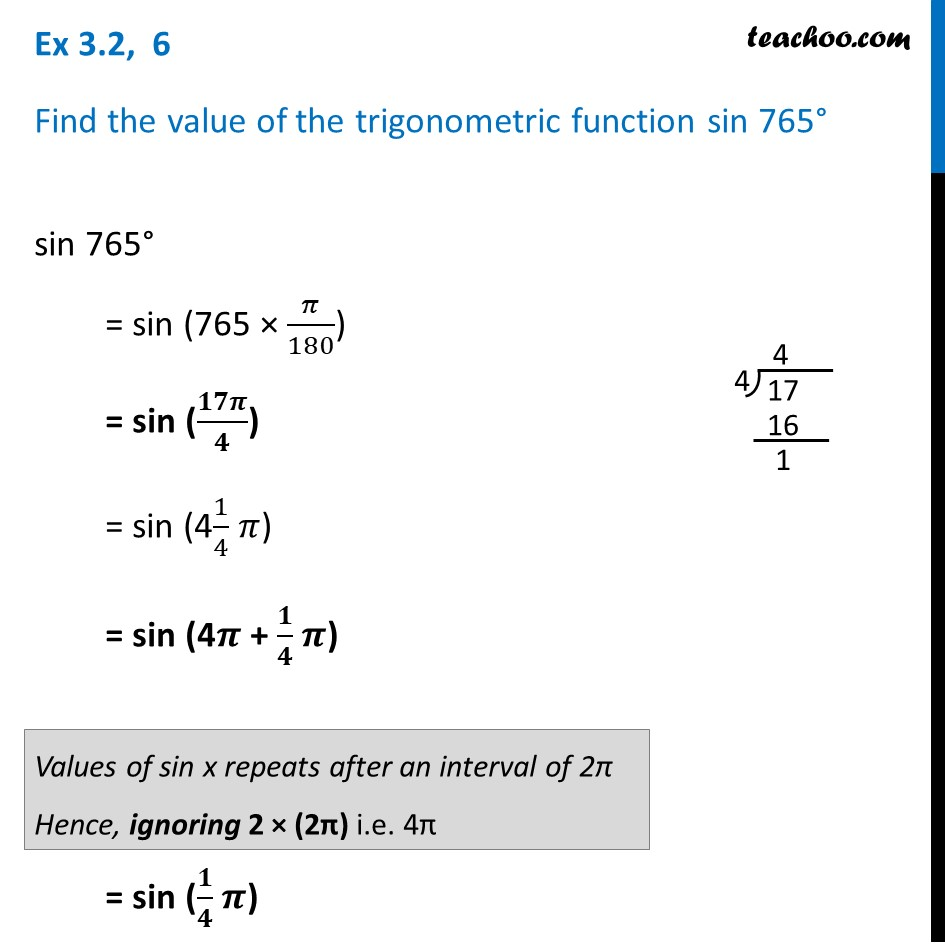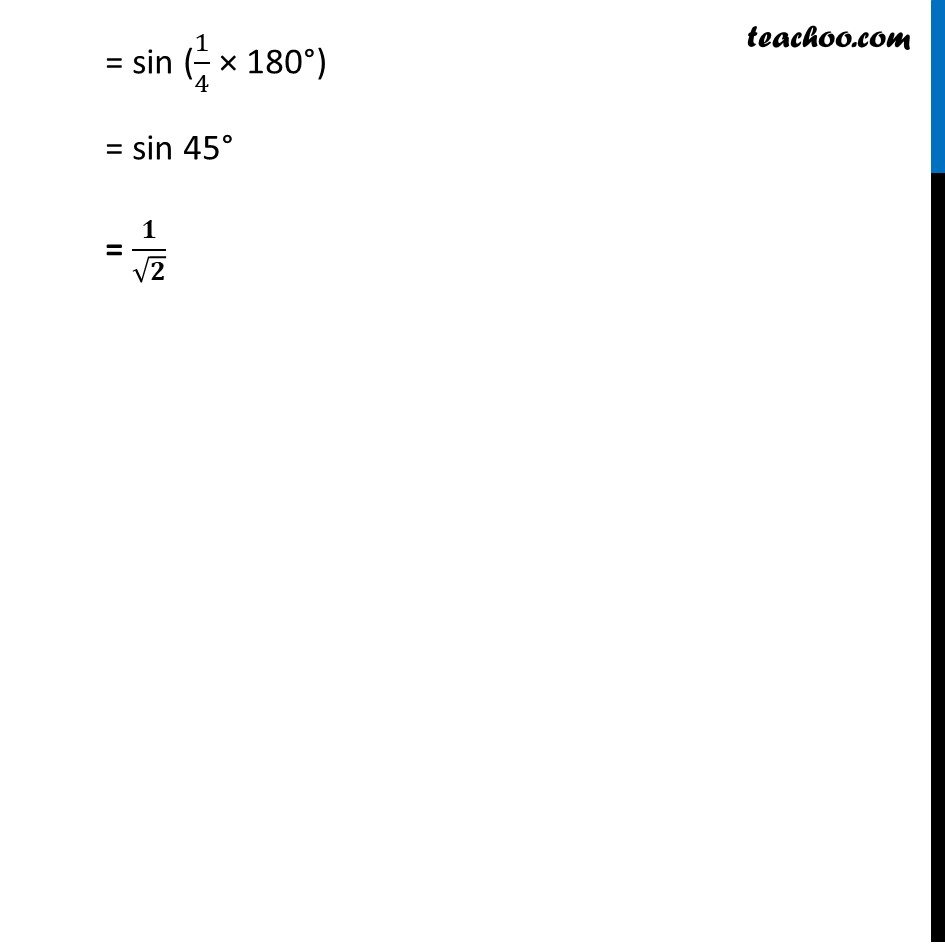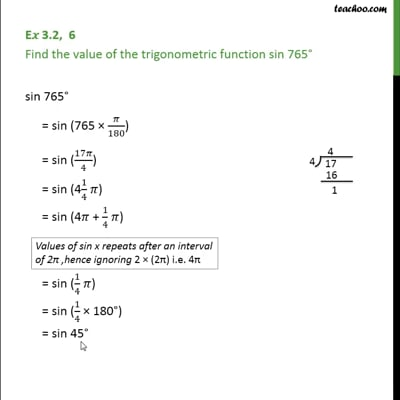Ex 3.2

Chapter 3 Class 11 Trigonometric Functions
Serial order wiseThis video is only available for Teachoo black users

Learn in your speed, with individual attention - Teachoo Maths 1-on-1 Class

### Transcript

Ex 3.2, 6 Find the value of the trigonometric function sin 765° sin 765° = sin (765 × 𝜋/180) = sin (𝟏𝟕𝝅/𝟒) = sin (41/4 𝜋) = sin (4𝝅 + 𝟏/𝟒 𝝅) = sin (𝟏/𝟒 𝝅) Values of sin x repeats after an interval of 2π Hence, ignoring 2 × (2π) i.e. 4π = sin (1/4 × 180°) = sin 45° = 𝟏/√𝟐